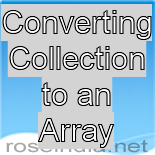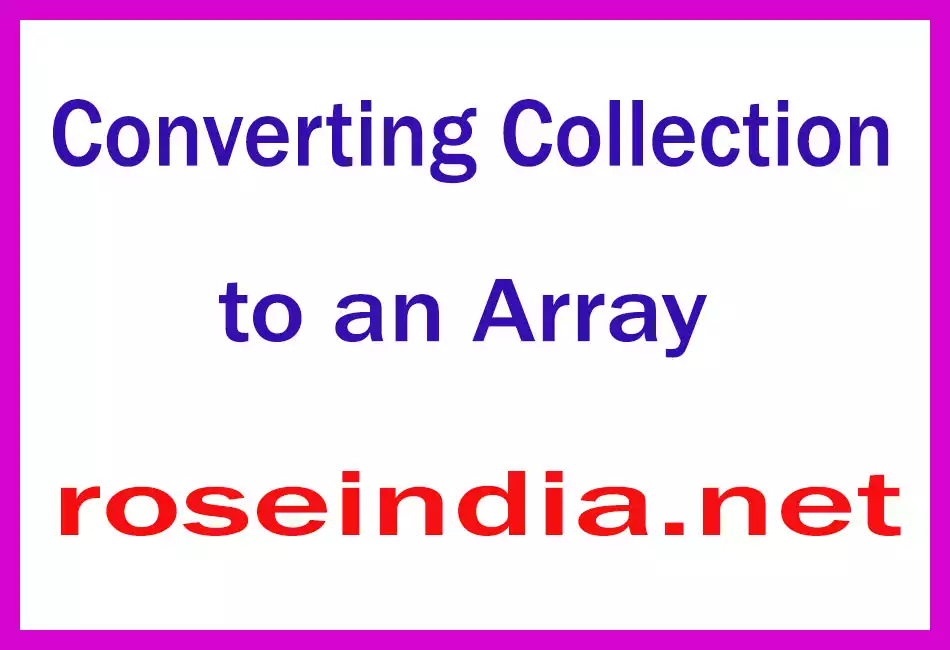# Converting Collection to an Array

Here is the illustration for the conversion from the collection to an array. In this section, you will learn how to do this.### Converting Collection to an Array

Here is the illustration for the conversion from the collection to an array. In this section, you will learn how to do this.# Converting Collection to an Array

Here is the illustration for the conversion from the collection to an array. In this section, you will learn how to do this. The given example gives you a brief introduction for convert collection to an array without losing any data or element present in the collection.

This program creates a List. List is a type of collection that contains a ordered list of elements. This list (collection) is converted into an array. Length of the created array is defined by the system according to the number of elements in the list. Each and every subscripts hold separate element.

Code Description:

Here is the explanation of some methods and APIs whatever has been used in the program:

List:
List
is the class of the java.util package which creates a list for containing some elements. In this program, this class is used with the type checking.

 ``` import java.util.*; public class CollectionToArray{   public static void main(String[] args){   List list = new ArrayList();   list.add("This ");    list.add("is ");    list.add("a ");   list.add("good ");   list.add("boy.");   String[] s1 = list.toArray(new String); //Collection to array    for(int i = 0; i < s1.length; ++i){   String contents = s1[i];   System.out.print(contents);   }    } }```